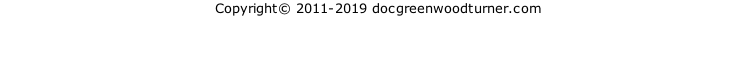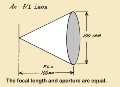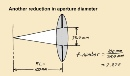﻿ Photo Exp Part 2Doc Green’s Woodturning SiteExposure Controls, Part 2

The Standard f-numbers

At the end of Part 1, we obtained this relationship:

f-number = focal length / aperture diameter

This section explains how the standard f-numbers are established in such a manner that switching from one to one next to it either doubles the light transmitted or cuts it in half.

Aperture diameter and f-numbers.   A lens with an adjustable aperture (diaphragm) can be set to any f-number (within its capability) simply by rotating the ring that controls the diaphragm.  The f-numbers are continuous over the range; they don’t go in clicks, not yet anyway.

Remember the factor-of-2 phenomenon at the beginning of this article?  A small change in the aperture will not make much difference as far as its effect on exposing the film is concerned. It takes a change that will either double or cut in half the amount of light entering the camera to make a noticeable difference.  And the way to do this is to either double, or cut in half, the area of the aperture through which the light passes.

What must you do to the diameter of a circle in order to double the area?  Multiply by 2?  No.  That would make the area 4 times greater because the area varies as the square of the diameter.

To double the area of a circle, multiply its diameter by the square root of 2, which is 1.414, rounded off.

Going in the other direction, what must happen to the diameter in order to cut the area in half?  And the answer is, . . .

To cut the area of a circle in half, divide the diameterby the square root of 2, or equivalently, multiply by 0.707.

What’s the point?  It is desirable to find a series of f-numbers so that in going from one to the next, you reduce the exposure by one half by reducing the area of the aperture by one half.  As it turns out, it’s not hard to do, but it does take a bit of arithmetic.  Press on.

Suppose we have an unusual lens, one whose diameter (maximum aperture) is the same as its focal length.  We’ll take the diameter and focal length to be 100 mm just to be specific. This represents a sizable chunk of glass because 100 mm is just about 4”.

At maximum aperture, what will be the f-number of this lens?

f-number = focal length / aperture diameter

= 100 mm / 100 mm= 1                      It’s an f/1 lens.

Let’s express the aperture diameter in terms of the f-number and focal length.  Here is the relationship, obtained by rearranging the definition of the f-number given above:

aperture diameter  =  focal length / f-number

To check this out, let’s apply it to our rather unusual lens:

aperture diameter   =  100 mm / 1

= 100 mm     We knew this.

Suppose we reduce the aperture diameter enough to cut the area, and light transmission, in half.  From the explanation above, we should divide the diameter by the square root of two, which is 1.414:

New aperture diameter = 100 mm / 1.414

= 70.7 mm

Calculate the f-number for this case:

f-number =  focal length / aperture diameter

=  100 mm / 70.7 mm=  1.414

What this tells us is that the f-number that will cut the f/1 exposure by one half is f/1.4.  If we take f/1 as the first in the series of standard f-numbers, then f/1.4 is the second in the series.

Let’s do another click, so to speak.  What should be the new aperture diameter that will reduce the exposure by one half from what it is with the 70.7 mm aperture?

To find the new diameter, divide the present one of 70.7 mm by 1.414, and you will get 50 mm.  To find the corresponding f-number,

f-number = focal length / aperture diameter

= 100 mm / 50 mm= 2

Thus, the third in our series of standard f-numbers is f/2.

Do another one.  Divide 50 mm by 1.414 to get the aperture that will transmit half as much light as the 50 mm aperture.  The result is 35.36 mm.  Then calculate the corresponding f-number:

f-number = focal length / aperture diameter

= 100 mm / 35.36 mm

= 2.828

This is f/2.8, with a little rounding off, and is the fourth inthe series of standard f-numbers.

Take a look at the series so far:    1,  1.4,   2,   2.8

What’s the relationship between these numbers?  If you have one, such as f/2, how do you get the next one?

Answer:  To get the next one in the series, multiply by 1.414.

The f-numbers are rounded off to two significant figures because more precision than this is not justified in light of the factor-of-2 phenomenon.

Here’s the complete series of standard f-numbers:

1,  1.4,  2,  2.8,  4,  5.6,  8,  11,  16,  22,  32,  45,  64,  90

And what is their significance?  In going from one to the one next to it, the exposure is either cut in half, if you go to a larger number, or doubled, if you go to a smaller number.

There is another relationship that allows you to calculate the standard f-numbers quickly.  Starting with 1, form a sequence where each number in the sequence is double the one before it:

1,  2,  4,  8,  16,  32,  64,  128,  256,  . . .

If you take the square root of each of these numbers, you get a standard f-number.  For example, the square root of 32 is 5.6, very nearly, and the square root of 64 is 8.

What’s an f-stop?  On most cameras, the ring that adjusts the aperture is provided with a detent (where it clicks into place) at each standard f-number.  That is, the ring tends to stop there. Hence the term, f-stop.  It’s a little like a bus stop, where the bus stops.  The terms f-number and f-stop are frequently used interchangeably.

top p2